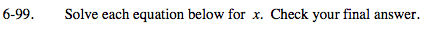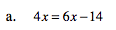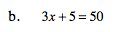### Home > MC2 > Chapter 6 > Lesson 6.2.3 > Problem6-99

6-99.Subtract 6x from both sides.

4x = 6x − 14
−6x −6x

−2x = −14

Divide each side by −2.

${\frac{-2\textit{x}}{-2}=\frac{-14}{-2}}$

x = 7

Try using the same method presented in (a).